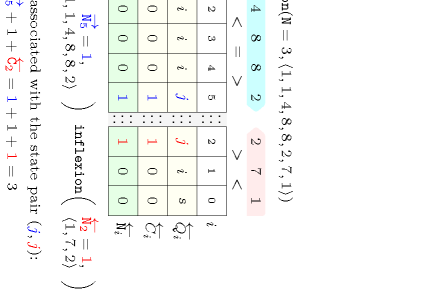## 5.193. inflexion

Origin

N. Beldiceanu

Constraint

$\mathrm{𝚒𝚗𝚏𝚕𝚎𝚡𝚒𝚘𝚗}\left(𝙽,\mathrm{𝚅𝙰𝚁𝙸𝙰𝙱𝙻𝙴𝚂}\right)$

Arguments
 $𝙽$ $\mathrm{𝚍𝚟𝚊𝚛}$ $\mathrm{𝚅𝙰𝚁𝙸𝙰𝙱𝙻𝙴𝚂}$ $\mathrm{𝚌𝚘𝚕𝚕𝚎𝚌𝚝𝚒𝚘𝚗}\left(\mathrm{𝚟𝚊𝚛}-\mathrm{𝚍𝚟𝚊𝚛}\right)$
Restrictions
 $𝙽\ge 0$ $𝙽\le \mathrm{𝚖𝚊𝚡}\left(0,|\mathrm{𝚅𝙰𝚁𝙸𝙰𝙱𝙻𝙴𝚂}|-2\right)$ $\mathrm{𝚛𝚎𝚚𝚞𝚒𝚛𝚎𝚍}$$\left(\mathrm{𝚅𝙰𝚁𝙸𝙰𝙱𝙻𝙴𝚂},\mathrm{𝚟𝚊𝚛}\right)$
Purpose

$𝙽$ is equal to the number of times that the following conjunctions of constraints hold:

• ${X}_{i}\mathrm{𝙲𝚃𝚁}{X}_{i+1}\wedge {X}_{i}\ne {X}_{i+1}$,

• ${X}_{i+1}={X}_{i+2}\wedge \cdots \wedge {X}_{j-2}={X}_{j-1}$,

• ${X}_{j-1}\ne {X}_{j}\wedge {X}_{j-1}¬\mathrm{𝙲𝚃𝚁}{X}_{j}$.

where ${X}_{k}$ is the ${k}^{th}$ item of the $\mathrm{𝚅𝙰𝚁𝙸𝙰𝙱𝙻𝙴𝚂}$ collection and $1\le i$, $i+2\le j$, $j\le n$ and $\mathrm{𝙲𝚃𝚁}$ is $<$ or $>$.

Example
 $\left(3,〈1,1,4,8,8,2,7,1〉\right)$ $\left(0,〈1,1,4,4,6,6,7,9〉\right)$ $\left(7,〈1,0,2,0,7,2,7,1,2〉\right)$

The first $\mathrm{𝚒𝚗𝚏𝚕𝚎𝚡𝚒𝚘𝚗}$ constraint holds since the sequence $11488271$ contains three inflexions peaks that respectively correspond to values 8, 2 and 7.

##### Figure 5.193.1. Illustration of the first example of the Example slot: a sequence of eight variables ${V}_{1}$, ${V}_{2}$, ${V}_{3}$, ${V}_{4}$, ${V}_{5}$, ${V}_{6}$, ${V}_{7}$, ${V}_{8}$ respectively fixed to values 1, 1, 4, 8, 8, 2, 7, 1 and its three inflexions in red, two peaks and one valley ($𝙽=3$)All solutions

Figure 5.193.2 gives all solutions to the following non ground instance of the $\mathrm{𝚒𝚗𝚏𝚕𝚎𝚡𝚒𝚘𝚗}$ constraint: $𝙽\in \left\{0,2\right\}$, ${V}_{1}=2$, ${V}_{2}\in \left[2,3\right]$, ${V}_{3}\in \left[1,2\right]$, ${V}_{4}\in \left[1,2\right]$, ${V}_{5}=3$, $\mathrm{𝚒𝚗𝚏𝚕𝚎𝚡𝚒𝚘𝚗}$$\left(𝙽,〈{V}_{1},{V}_{2},{V}_{3},{V}_{4},{V}_{5}〉\right)$.

##### Figure 5.193.2. All solutions corresponding to the non ground example of the $\mathrm{𝚒𝚗𝚏𝚕𝚎𝚡𝚒𝚘𝚗}$ constraint of the All solutions slot where each inflexion (i.e. peak or valley) is coloured in orange or cyan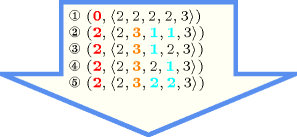Typical
 $𝙽>0$ $|\mathrm{𝚅𝙰𝚁𝙸𝙰𝙱𝙻𝙴𝚂}|>2$ $\mathrm{𝚛𝚊𝚗𝚐𝚎}$$\left(\mathrm{𝚅𝙰𝚁𝙸𝙰𝙱𝙻𝙴𝚂}.\mathrm{𝚟𝚊𝚛}\right)>1$
Symmetries
• Items of $\mathrm{𝚅𝙰𝚁𝙸𝙰𝙱𝙻𝙴𝚂}$ can be reversed.

• One and the same constant can be added to the $\mathrm{𝚟𝚊𝚛}$ attribute of all items of $\mathrm{𝚅𝙰𝚁𝙸𝙰𝙱𝙻𝙴𝚂}$.

Arg. properties

Functional dependency: $𝙽$ determined by $\mathrm{𝚅𝙰𝚁𝙸𝙰𝙱𝙻𝙴𝚂}$.

Usage

Useful for constraining the number of inflexions of a sequence of domain variables.

Remark

Since the arity of the arc constraint is not fixed, the $\mathrm{𝚒𝚗𝚏𝚕𝚎𝚡𝚒𝚘𝚗}$ constraint cannot be currently described with the graph-based representation. However, this would not hold anymore if we were introducing a slot that specifies how to merge adjacent vertices of the final graph.

Counting
 Length ($n$) 2 3 4 5 6 7 8 Solutions 9 64 625 7776 117649 2097152 43046721

Number of solutions for $\mathrm{𝚒𝚗𝚏𝚕𝚎𝚡𝚒𝚘𝚗}$: domains $0..n$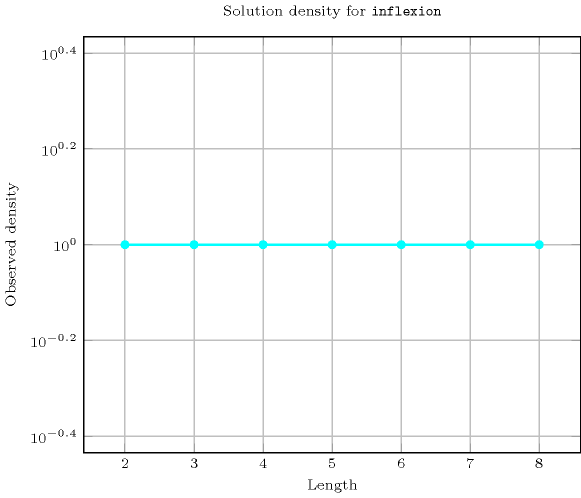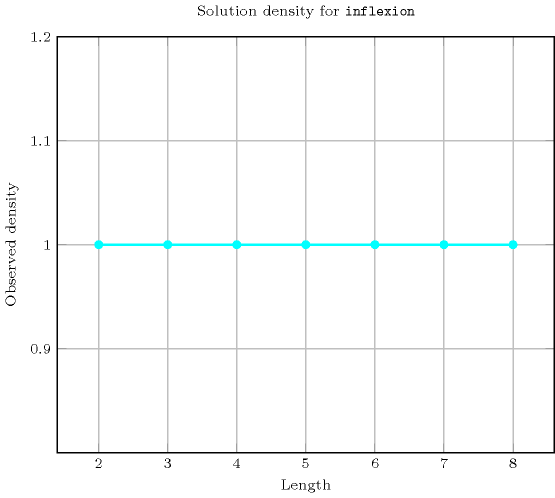Length ($n$)2345678
Total9646257776117649209715243046721
 Parameter value

09361354981841685625731
1-28320258818494125284828120
2--1703348440584923205069970
3---13424044677893612341184
4----1281054915214547186
5-----1446048354520
6------1880010

Solution count for $\mathrm{𝚒𝚗𝚏𝚕𝚎𝚡𝚒𝚘𝚗}$: domains $0..n$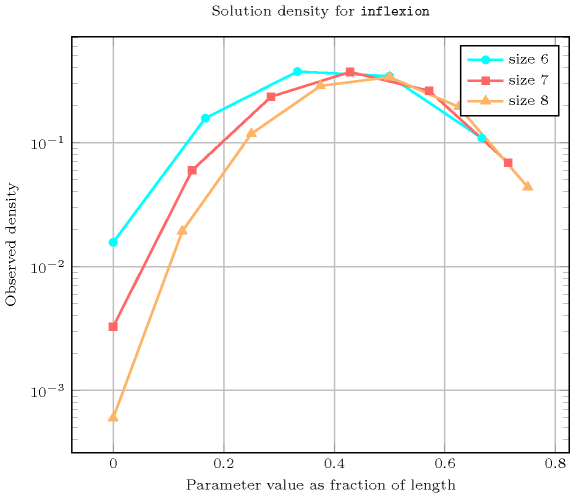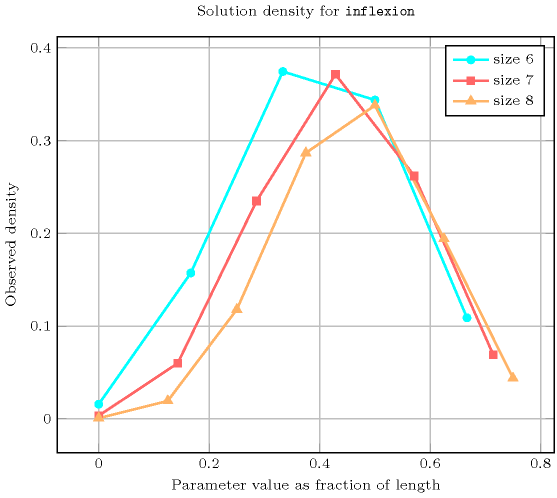Keywords
Cond. implications

$•$ $\mathrm{𝚒𝚗𝚏𝚕𝚎𝚡𝚒𝚘𝚗}\left(𝙽,\mathrm{𝚅𝙰𝚁𝙸𝙰𝙱𝙻𝙴𝚂}\right)$

with  $𝙽>0$

implies $\mathrm{𝚊𝚝𝚕𝚎𝚊𝚜𝚝}_\mathrm{𝚗𝚟𝚊𝚕𝚞𝚎}$$\left(\mathrm{𝙽𝚅𝙰𝙻},\mathrm{𝚅𝙰𝚁𝙸𝙰𝙱𝙻𝙴𝚂}\right)$

when  $\mathrm{𝙽𝚅𝙰𝙻}=2$.

$•$ $\mathrm{𝚒𝚗𝚏𝚕𝚎𝚡𝚒𝚘𝚗}\left(𝙽,\mathrm{𝚅𝙰𝚁𝙸𝙰𝙱𝙻𝙴𝚂}\right)$

with  $\mathrm{𝚟𝚊𝚕𝚕𝚎𝚢}$$\left(\mathrm{𝚅𝙰𝚁𝙸𝙰𝙱𝙻𝙴𝚂}.\mathrm{𝚟𝚊𝚛}\right)=0$

implies $\mathrm{𝚙𝚎𝚊𝚔}$$\left(𝙽,\mathrm{𝚅𝙰𝚁𝙸𝙰𝙱𝙻𝙴𝚂}\right)$.

$•$ $\mathrm{𝚒𝚗𝚏𝚕𝚎𝚡𝚒𝚘𝚗}\left(𝙽,\mathrm{𝚅𝙰𝚁𝙸𝙰𝙱𝙻𝙴𝚂}\right)$

with  $\mathrm{𝚙𝚎𝚊𝚔}$$\left(\mathrm{𝚅𝙰𝚁𝙸𝙰𝙱𝙻𝙴𝚂}.\mathrm{𝚟𝚊𝚛}\right)=0$

implies $\mathrm{𝚟𝚊𝚕𝚕𝚎𝚢}$$\left(𝙽,\mathrm{𝚅𝙰𝚁𝙸𝙰𝙱𝙻𝙴𝚂}\right)$.

Automaton

Figure 5.193.3 depicts the automaton associated with the $\mathrm{𝚒𝚗𝚏𝚕𝚎𝚡𝚒𝚘𝚗}$ constraint. To each pair of consecutive variables $\left({\mathrm{𝚅𝙰𝚁}}_{i},{\mathrm{𝚅𝙰𝚁}}_{i+1}\right)$ of the collection $\mathrm{𝚅𝙰𝚁𝙸𝙰𝙱𝙻𝙴𝚂}$ corresponds a signature variable ${S}_{i}$. The following signature constraint links ${\mathrm{𝚅𝙰𝚁}}_{i}$, ${\mathrm{𝚅𝙰𝚁}}_{i+1}$ and ${S}_{i}$: $\left({\mathrm{𝚅𝙰𝚁}}_{i}<{\mathrm{𝚅𝙰𝚁}}_{i+1}⇔{S}_{i}=0\right)\wedge \left({\mathrm{𝚅𝙰𝚁}}_{i}={\mathrm{𝚅𝙰𝚁}}_{i+1}⇔{S}_{i}=1\right)\wedge \left({\mathrm{𝚅𝙰𝚁}}_{i}>{\mathrm{𝚅𝙰𝚁}}_{i+1}⇔{S}_{i}=2\right)$.

##### Figure 5.193.3. Automaton of the $\mathrm{𝚒𝚗𝚏𝚕𝚎𝚡𝚒𝚘𝚗}$ constraint (state $s$ means that we are in stationary mode, state $i$ means that we are in increasing mode, state $j$ means that we are in decreasing mode, a new inflexion is detected each time we switch from increasing to decreasing mode – or conversely from decreasing to increasing mode – and the counter $C$ is incremented accordingly)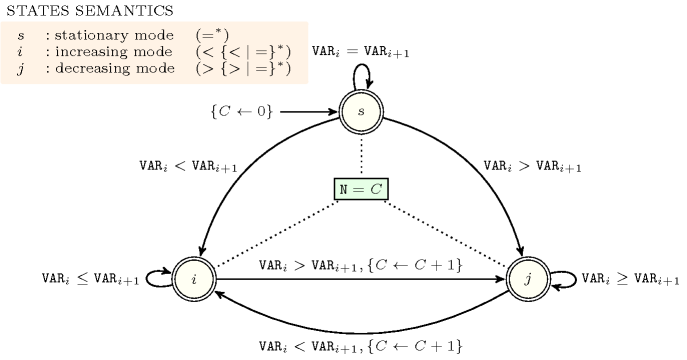##### Figure 5.193.4. Hypergraph of the reformulation corresponding to the automaton of the $\mathrm{𝚒𝚗𝚏𝚕𝚎𝚡𝚒𝚘𝚗}$ constraint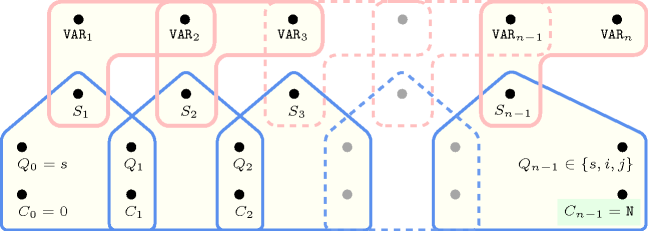##### Figure 5.193.5. Glue matrix associated with the automaton of the $\mathrm{𝚒𝚗𝚏𝚕𝚎𝚡𝚒𝚘𝚗}$ constraint
 $s$ $\left({=}^{*}\right)$ $i$ $\left(<{\left\{<|=\right\}}^{*}\right)$ $j$ $\left(>{\left\{>|=\right\}}^{*}\right)$ $s$ $\left({=}^{*}\right)$ 0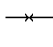$\stackrel{←}{C}$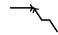$\stackrel{←}{C}$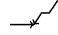$i$ $\left(<{\left\{<|=\right\}}^{*}\right)$ $\stackrel{\to }{C}$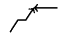$\stackrel{\to }{C}+1+\stackrel{←}{C}$$\stackrel{\to }{C}+\stackrel{←}{C}$$j$ $\left(>{\left\{>|=\right\}}^{*}\right)$ $\stackrel{\to }{C}$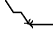$\stackrel{\to }{C}+\stackrel{←}{C}$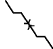$\stackrel{\to }{C}+1+\stackrel{←}{C}$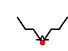##### Figure 5.193.6. Illustrating the use of the state pair $\left(j,j\right)$ of the glue matrix for linking $𝙽$ with the counters variables obtained after reading the prefix $1,1,4,8,8,2$ and corresponding suffix $2,7,1$ of the sequence $1,1,4,8,8,2,7,1$; note that the suffix $2,7,1$ (in pink) is proceed in reverse order; the left (resp. right) table shows the initialisation (for $i=0$) and the evolution (for $i>0$) of the state of the automaton and its counter $C$ upon reading the prefix $1,1,4,8,8,2$ (resp. the reverse suffix $1,7,2$).